# SAT Math Multiple Choice Question 236: Answer and Explanation

### Test Information

Question: 236

11. y = x2 - 2x
y = 2x - 1

This system has two solutions for (x, y). What is the larger value of x ?

• A. 2-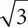• B.• C. 2 +• D. 5

C Start by setting the two equations equal to each other to get x2 - 2x = 2x - 1. Manipulate the equation into the ax2 + bx + c = 0 form, which is x2 - 4x + 1 = 0. Use the quadratic equation, which states that x =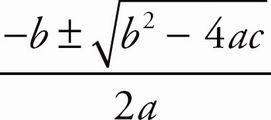to get x =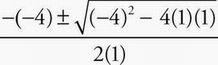. Solve for x to get x =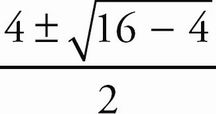, then x =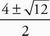or x =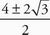, so x = 2 ±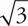. The greater of the two possible values for x is x = 2 +. Therefore, the correct answer is (C).